# SBI PO Prelims Reasoning Ability Quiz – 21

## SBI PO Prelims Reasoning Ability Quiz

Almost all major competitive exams in the country have a section on Reasoning Ability, and it is also one of the most scoring sections. Candidates can ace this section in exams if they practice regularly in a dedicated manner. So here we are providing you with the SBI PO Prelims Reasoning Ability Quiz to help you prepare better. This SBI PO Prelims Reasoning Ability Quiz includes all of the most recent pattern-based questions, as well as Previous Year Questions. This SBI PO Prelims Reasoning Ability Quiz is totally FREE. Candidates will be provided with a detailed explanation of each question in this SBI PO Prelims Reasoning Ability Quiz. Candidates must practice this SBI PO Prelims Reasoning Ability Quiz to achieve a good score in the Reasoning Ability Section.

Directions (1-5): Each of the questions given below consists of a question and two statements numbered I and II. You have to decide whether the data provided in the statements are sufficient to answer the question.

1. Conclusion: Some Milk are Coffee. Some Coffee are not Cold-drinks. Which of the following set of statement is required that logically satisfies given conclusions (Given statements to be true even if they seem to be at variance from commonly known facts).
Statement I: Some Milk are Drink. Some Coffee are Tea.
Statement II: All drink are Coffee. No Tea is Cold-drink.
(a) If statement I alone is sufficient to answer the question, but statement II by itself is not sufficient to answer the question.
(b) If statement II by itself is sufficient to answer the question, but statement I alone is not sufficient to answer the question.
(c) If statement either I or II is sufficient to answer the question.
(d) If both the statements I and II taken together are not sufficient to answer the question.
(e) If both the statements I and II taken together are sufficient to answer the question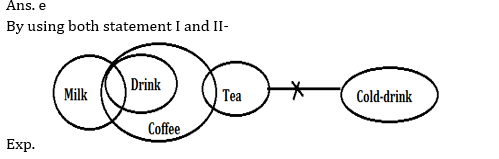/su_spoiler]

2. Six persons A, B, C, D, E and F are sitting around a circular table. Are all facing towards the center?
Statement I: E sits second to the left of A, who is facing towards the center. C sits opposite to D. F sits to the immediate right of A. A sit second left of C and C does not sit between A and E. F sits second to right of D. F sits third left of E.
Statement II: F sits second left of B. E sits second right of C and is not an immediate neighbour of F. D sits third left of C. B is facing towards the center.
(a) If statement I alone is sufficient to answer the question, but statement II by itself is not sufficient to answer the question.
(b) If statement II by itself is sufficient to answer the question, but statement I alone is not sufficient to answer the question.
(c) If statement either I or II is sufficient to answer the question.
(d) If both the statements I and II taken together are not sufficient to answer the question.
(e) If both the statements I and II taken together are sufficient to answer the question.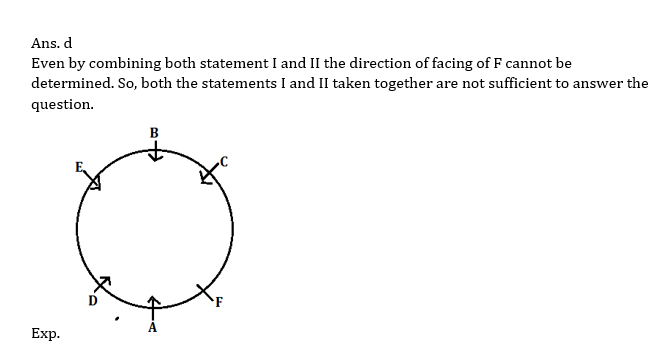/su_spoiler]

3. What is code of ‘Allegation’?
Statement I: In certain code language, ‘Speech audience popularity’ is coded as- ‘kl nu bp’ and ‘Conference debate report’ is coded as- ‘nu ga tr’
Statement II: In certain code language, ‘Deliver patience Speech’ is coded as- ‘bt hd kl’ and ‘Allegation conference popularity’ is coded as- ‘wa ga bp’.
(a) If statement I alone is sufficient to answer the question, but statement II by itself is not sufficient to answer the question.
(b) If statement II by itself is sufficient to answer the question, but statement I alone is not sufficient to answer the question.
(c) If statement either I or II is sufficient to answer the question.
(d) If both the statements I and II taken together are not sufficient to answer the question.
(e) If both the statements I and II taken together are sufficient to answer the question.

Ans. e
Exp. By using both statement I and II the code of Allegation will be- wa./su_spoiler]

4. Five persons P, Q, R, S, T are sitting in a row facing north direction. Who among the following sits at extreme left end?
Statement I: Q is third to the left of P. T is not an immediate neighbour of P. S sits second to the right of R.
Statement II: T sits second to the left of P. More than two persons sit between Q and S. Q sits to the left of R.
(a) If statement I alone is sufficient to answer the question, but statement II by itself is not sufficient to answer the question.
(b) If statement II by itself is sufficient to answer the question, but statement I alone is not sufficient to answer the question.
(c) If statement either I or II is sufficient to answer the question.
(d) If both the statements I and II taken together are not sufficient to answer the question.
(e) If both the statements I and II taken together are sufficient to answer the question.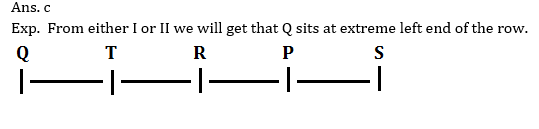/su_spoiler]

5. Who among the following is the father of K?
I. L is the father of R. R is the brother-in-law of D. D is the wife of K. K is the son of M, who is the wife of L.
II. F is the son of K. F is married to G, who is the daughter-in-law of D. F is the grandson of M.
(a) If statement I alone is sufficient to answer the question, but statement II by itself is not sufficient to answer the question.
(b) If statement II by itself is sufficient to answer the question, but statement I alone is not sufficient to answer the question.
(c) If statement either I or II is sufficient to answer the question.
(d) If both the statements I and II taken together are not sufficient to answer the question.
(e) If both the statements I and II taken together are sufficient to answer the question.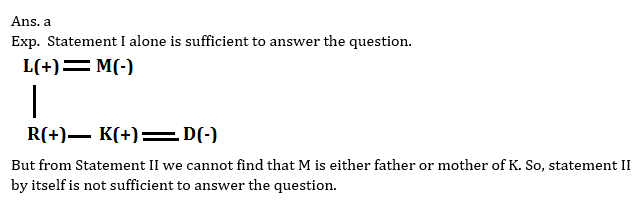/su_spoiler]

Directions (6-10): Read the following information carefully and answer the questions given below it:
Some boxes are arranged one above another. Box R is immediately above box M, which is of blue color. Only two boxes are there in between orange box and box T. Orange box is above box T. There is only one box in between box P and box S. Box S is of purple color and is placed above box R. There are three boxes in between orange box and green box. Green box is placed below T. Only one box is placed in between box R and Orange box. More than four boxes are placed in between box P and Orange box. Not more than two boxes are placed in between box M and box S.
6. How many boxes are there in the arrangement?
(a)Ten
(b)Eleven
(c) Fourteen
(d) Twelve
(e) None of these

Ans. b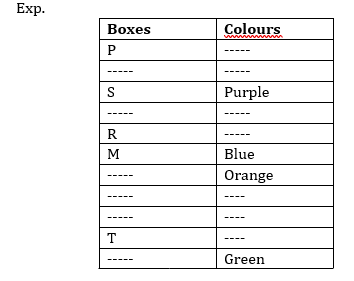/su_spoiler]

7. How many boxes are there between box R and box T?
(a)Five
(b)One
(c) Four
(d) Two
(e) None of these

Ans. c/su_spoiler]

8. Which box is immediately above orange box?
(a) S
(b)R
(c) Purple box
(d)M
(e) Green box

Ans. d/su_spoiler]

9. Which box is placed at top and bottom respectively?
(a) T, S
(b)R, Purple box
(c) P, Green box
(d)T, M
(e) Blue box, R

Ans. c/su_spoiler]

10. How many boxes are there between purple box and Green box?
(a)Ten
(b)Eight
(c) Seven
(d) Six
(e) None of these

Ans. c/su_spoiler]

### Preparation Kit PDF

#### Most important PDF’s for Bank, SSC, Railway and Other Government Exam : Download PDF Now

AATMA-NIRBHAR Series- Static GK/Awareness Practice Ebook PDF Get PDF here
The Banking Awareness 500 MCQs E-book| Bilingual (Hindi + English) Get PDF here
AATMA-NIRBHAR Series- Banking Awareness Practice Ebook PDF Get PDF here
Computer Awareness Capsule 2.O Get PDF here
AATMA-NIRBHAR Series Quantitative Aptitude Topic-Wise PDF 2020 Get PDF here
Memory Based Puzzle E-book | 2016-19 Exams Covered Get PDF here
Caselet Data Interpretation 200 Questions Get PDF here
Puzzle & Seating Arrangement E-Book for BANK PO MAINS (Vol-1) Get PDF here
3